# Inductive Conformal Predictor

Inductive conformal predictors (ICPs) is a modification of conformal predictors in order to improve the computational efficiency for large data sets. They are also know as "split conformal predictors".

## Definition

An inductive conformal predictor (ICM) determined by a conformity measure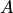and an ascending sequence of positive integers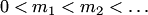is a confidence predictor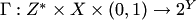(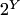is a set of all subsets of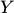) such that the prediction sets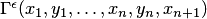are computed as follows:

• if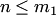, set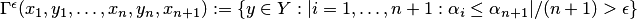,

where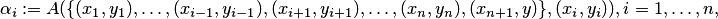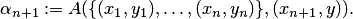• otherwise, find thesuch that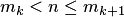and set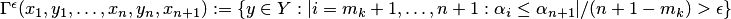,

where the nonconformity scores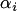are defined by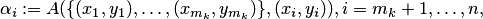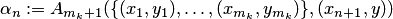and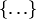designates the bag (multiset) of observations.

The standard assumption for ICPs as well as for conformal predictors is randomness assumption (also called the i.i.d. assumption).

Inductive conformal predictors can be generalized by Mondrian conformal predictors to a wider class of confidence predictors. Conformal predictors can be considered as an important special case of ICPs.

## Desiderata

### Validity

All the statements in the section are given under the randomness assumption.

Smoothed ICPs are defined analogously to smoothed conformal predictors:is set to the set of all labels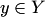such that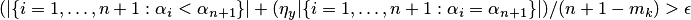,

where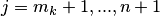, the conformity scoresare defined as before and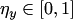is a random number.

As in the case of conformal predictors, we can formulate analogous statements for ICPs using the same terminology.

Theorem All smoothed ICPs are exactly valid.

Corollary All smoothed ICPs are asymptotically exact.

Corollary All ICPs are conservatively valid.

Corollary All ICPs are asymptotically conservative.

To put it simply, in the long run the frequency of erroneous predictions given by ICPs does not exceedat each confidence level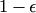.

### Efficiency

As inductive conformal predictors are automatically valid, the main goal is to improve their efficiency: to make the prediction sets ICPs output as small as possible. See criteria of efficiency.

In comparison with corresponding conformal predictors determined by the same non-conformity measure, ICPs are inferior in terms of predictive efficiency (however, the loss in predictive efficiency appears to be small), but outperform the CPs in computational efficiency.